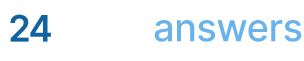# Probability

## Homework Help & Tutoring

We offer an array of different online Probability tutors, all of whom are advanced in their fields and highly qualified to instruct you.Send your subject help request Submit your homework problem, or a general tutoring request.
Get quotes from qualified tutors Receive a response from one of our tutors as soon as possible, sometimes within minutes!
Probability Tutors Available Now
96 tutors available
Probability Homework Library
243 total solutions
See what our students are saying
Can you help me with my homework in less than 24 hours?
Can you help me with my exam/quiz/test?
How much will it cost?
What kind of payments do you accept?

# Probability

Probability is a way of determining how likely something is to happen, and the area of math called probability theory gives us the mathematical tools necessary to analyze each situation and perform the necessary calculations. Probability is used extensively in statistics, finance, gambling, science, and philosophy.

A rigorous course in probability will cover many of the topics listed below:

• The need for measure theory
• Probability triples
• Probabilistic foundations
• Expected values
• Inequalities and convergence
• Distributions of random variables
• Stochastic processes and gambling games
• Discrete Markov chains
• Probability theorems
• Weak convergence
• Characteristic functions
• Decomposition of probability laws
• Conditional probability and expectation
• Martingales
• General stochastic processes

A large number of good books on probability can be found; a good place to start being Amazon.com. In addition, there is an excellent probability tutorial at West Texas A&M University.

## College Probability Homework Help

Since we have tutors in all Probability related topics, we can provide a range of different services. Our online Probability tutors will:

• Provide specific insight for homework assignments.
• Review broad conceptual ideas and chapters.
• Simplify complex topics into digestible pieces of information.
• Answer any Probability related questions.
• Tailor instruction to fit your style of learning.

With these capabilities, our college Probability tutors will give you the tools you need to gain a comprehensive knowledge of Probability you can use in future courses.